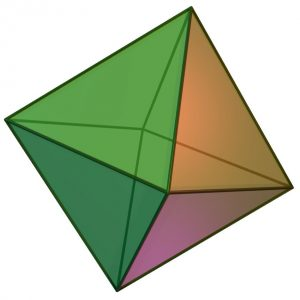GFG App
Open AppBrowser
Continue

# Program to calculate volume of Octahedron

Given the side of the Octahedron then calculate the volume of Octahedron.
Examples:

```Input : 3
Output : 12.7279

Input : 7
Output : 161.692```

A regular Octahedron has eight faces,twelve edges and six vertices. It has eight triangles with edges of equal length and effectively two square pyramids meeting at their bases.Image Source : Wikimedia

Properties of Octahedron:
Number of faces: 8
Number of edges: 12
Number of vertices: 6
Volume = √2/3 × a3 where a is the side of Octahedron

## CPP

 `// CPP Program to calculate  ` `// volume of Octahedron ` `#include ` `using` `namespace` `std; ` ` `  `// utility Function ` `double` `vol_of_octahedron(``double` `side) ` `{ ` `    ``return` `((side*side*side)*(``sqrt``(2)/3)); ` `} ` ` `  `// Driver Function ` `int` `main() ` `{ ` `    ``double` `side = 3; ` `    ``cout << ``"Volume of octahedron ="` `         ``<< vol_of_octahedron(side) ` `         ``<< endl; ` `} `

## Java

 `// Java Program to calculate  ` `// volume of Octahedron ` ` `  `import` `java.io.*; ` `class` `GFG  ` `{ ` `    ``public` `static` `void` `main (String[] args)  ` `      ``{ ` `          ``// Driver Function ` `         ``double` `side = ``3``; ` `        ``System.out.print(``"Volume of octahedron = "``); ` `        ``System.out.println(vol_of_octahedron(side)); ` ` `  `      ``} ` ` `  `    ``// utility Function ` `    ``static` `double` `vol_of_octahedron(``double` `side) ` `      ``{ ` `         ``return` `((side*side*side)*(Math.sqrt(``2``)/``3``)); ` `      ``} ` `} ` ` `  `// This code is contributed ` `// by Azkia Anam. `

## Python3

 `# Python3 Program to calculate  ` `# volume of Octahedron ` ` `  `import` `math ` ` `  `# utility Function ` `def` `vol_of_octahedron(side): ` `    ``return` `((side``*``side``*``side)``*``(math.sqrt(``2``)``/``3``)) ` ` `  `# Driver Function ` `side ``=` `3` `print``(``"Volume of octahedron ="``, ` `      ``round``(vol_of_octahedron(side),``4``)) ` ` `  `# This code is contributed ` `# by Azkia Anam. `

## C#

 `// C# Program to calculate  ` `// volume of Octahedron ` `using` `System; ` ` `  `class` `GFG  ` `{ ` `    ``public` `static` `void` `Main ()  ` `    ``{ ` `        ``// Driver Function ` `        ``double` `side = 3; ` `        ``Console.Write(``"Volume of octahedron = "``); ` `        ``Console.WriteLine(vol_of_octahedron(side)); ` ` `  `    ``} ` ` `  `    ``// utility Function ` `    ``static` `double` `vol_of_octahedron(``double` `side) ` `    ``{ ` `        ``return` `((side*side*side)*(Math.Sqrt(2)/3)); ` `    ``} ` `} ` ` `  `// This code is contributed ` `// by vt_m. `

## PHP

 ` `

## Javascript

 ` `

Output:

` Volume of octahedron = 12.7279`

Time complexity : O(1)
Auxiliary Space : O(1)

My Personal Notes arrow_drop_up